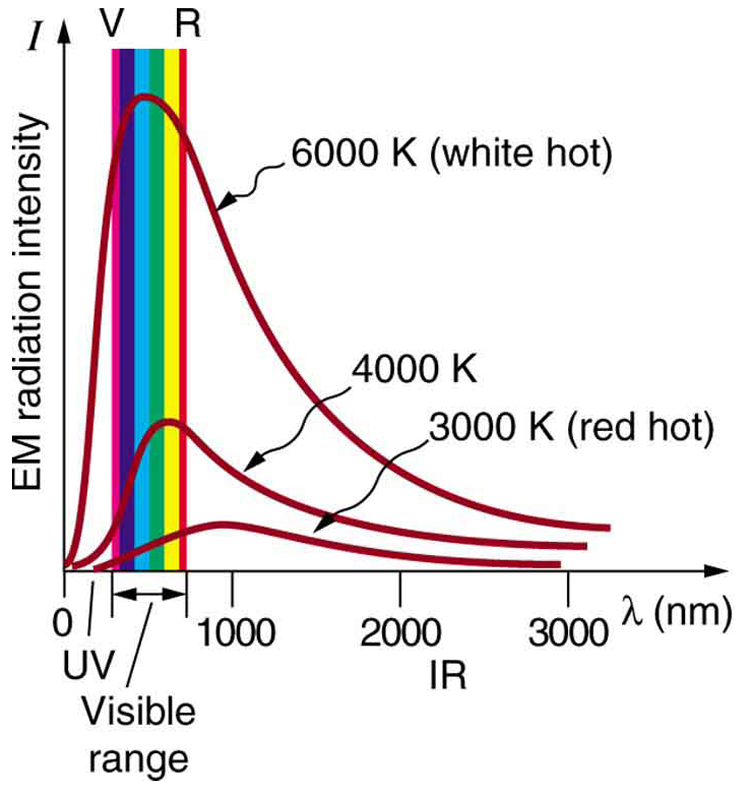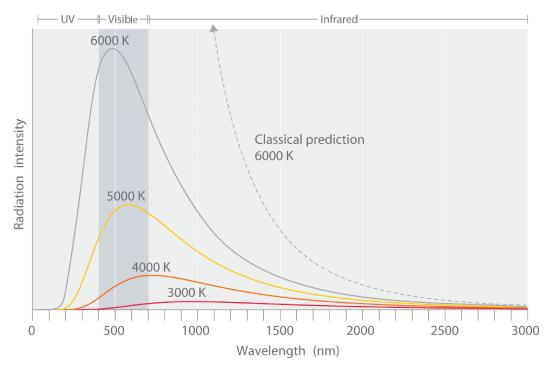# Quantization of Energy Study Guide

INTRODUCTION

Have you ever wondered about the small differences in the location of the electrons? How are the charges shifting? Everything is linked with quantum theory. Max Planck, a German physicist, studied the emission of lights by hot objects. Why this phenomenon occurs, all depends upon the quantization of energy.

### QUANTIZATION OF ENERGY- DEFINITION

An electron can radiate or absorb energy as radiation only in limited amounts or bundles called quanta. This is known as the quantization of energy. Quantization is a physical quantity that only has discrete values. The energy levels of electrons of metals can be quantized.Source

### WHAT IS QUANTA?

Quanta can be defined as the minimum quantity of energy that can either be gained or lost by an electron. Hence, if you see the energy levels of an electron, you can see that an atom needs to gain or lose energy to reach the next level or fall to the level. Absorption of heat or light helps the electrons gain energy to go to the next energy level or fall to lower energy levels.Source

### FORMULA

Through the experiment of emission of light from hot objects, Planck devised a formula. He showed that the quantity of radiant energy emitted or absorbed by an object is directly proportional to the frequency of the radiation.

``                                     E = h v``

Where,

E = Energy of quantum radiation, in joules

v = Frequency

h = A fundamental constant called Planck’s constant

The value of Planck’s constant is taken as h = 6.626 × 10⁻³⁴ J⋅s. The huge energy change results in emission or absorption of high frequency, whereas the small energy changes result in low emission or absorption of frequency. The energy present in a system must decrease or increase in the units of h x v.

### EXAMPLE PROBLEM

What is the energy of a photon of green light with a frequency of 8.65 × 10¹⁴ Hz?

Frequency (v) = 8.65 × 10¹⁴

Planck’s Constant (h) = 6.626 × 10⁻³⁴ J⋅s

∴ E = h v

or, E = 6.626 × 10⁻³⁴ x 8.65 × 10¹⁴

or, E = 5.732 x 10⁻¹⁹ J

The energy of a photon of light is 5.732 x 10⁻¹⁹ J.

### FAQs:

1. What is meant by quantization of energy?

An electron can radiate or absorb energy as radiations only in limited amounts or bundles called quanta. This is known as the quantization of energy.

2. What is meant by quantization?

Quantization in physics is the method of transition from an understanding of physical phenomena to a newer understanding, is known as “quantum mechanics.”

3. What is the cause of the quantization of energy?

The quantization of electric charge is the property under which all free charges are integral multiples of the basic unit of charge of an electron or proton. The primary cause of quantization is that only an integral number of electrons can be transmitted from one body to another on rubbing.

4. What is an example of quantized energy?

Some examples of quantized energy are the tire size, speedboat waves, harp, etc.

*We hope you enjoyed studying this lesson and learned something cool about the Quantization of Energy! Join our Discord community to get any questions you may have answered and to engage with other students just like you! Don’t forget to download our App to experience our fun VR classrooms – we promise it makes studying much more fun! *😎

## SOURCES:

1. Quantization of Energy: https://www.ck12.org/c/chemistry/quantization-of-energy/lesson/Quantization-of-Energy-CHEM/. Accessed 7th March 2022.
2. Quantization of Energy: https://courses.lumenlearning.com/physics/chapter/29-1-quantization-of-energy/. Accessed 7th March 2022.
]]>